CATEGORIES:

# Tangents to an Ellipse

Consider the equation of an ellipse: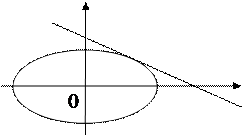у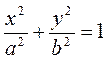.

М000)

х

As is known, the equation of a tangent to a curve is determined by the formula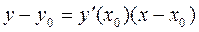.

Differentiating the equation of the ellipse as an implicit function, we obtain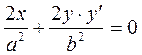, whence, or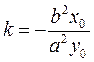.

Substituting this k, we find the equation of the tangent line: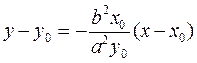.

Let us transform it:,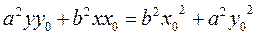. Dividing by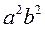, we obtain.

Since the point М0 belongs to the ellipse, the coordinates of М0 must satisfy its equation, and the right-hand side equals one.

Thus, the equation of a tangent to an ellipse is. (18)

Example. Given the ellipse given, find the distance between its foci, eccentricity, and the equations of directrices.

Let us reduce the equation it to the classical form (17):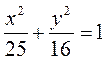;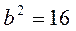;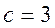,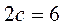.

Let us find the eccentricity: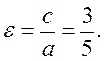The equations of the directrices are,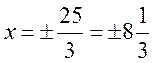.

Hyperbola

Definition. The locus of the points for which the difference of distances to two fixed points is constant equal to is called a hyperbola.

As for an ellipse, we introduce a new coordinate system: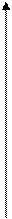уМ(х,у)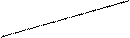r1 r2F1(–c,0) M1 M2 F2(c,0) x

To derive the equation of a hyperbola, we take an arbitrary point М(х,у) on the hyperbola and consider the distances from this point to the foci: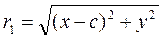;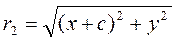.

The characteristic feature of the line is, by definition,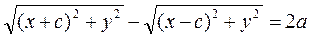.

We have composed an equation of the hyperbola. Let us reduce to a convenient form (by analogy with the ellipse):.

We divide both sides by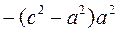:Changing the sign, we obtain the equation of a hyperbola: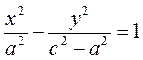.

Since 2a<2c, we denote the difference of squares by. (**)

Thus, we have obtained the classical equation of a hyperbola:. (19)

Date: 2015-01-02; view: 1130

 <== previous page | next page ==> The eccentricity and directrix of an ellipse. Consider the focal radii of an ellipse | Asymptotes of a Hyperbola
doclecture.net - lectures - 2014-2023 year. Copyright infringement or personal data (0.007 sec.)